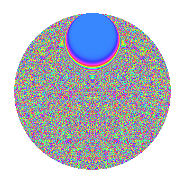# Properties

 Label 1849.2.oLevel 1849 Weight 2 Character orbit o Rep. character $$\chi_{1849}(9,\cdot)$$ Character field $$\Q(\zeta_{903})$$ Dimension 79128 Sturm bound 315

# Related objects

## Defining parameters

 Level: $$N$$ $$=$$ $$1849 = 43^{2}$$ Weight: $$k$$ $$=$$ $$2$$ Character orbit: $$[\chi]$$ $$=$$ 1849.o (of order $$903$$ and degree $$504$$) Character conductor: $$\operatorname{cond}(\chi)$$ $$=$$ $$1849$$ Character field: $$\Q(\zeta_{903})$$ Sturm bound: $$315$$

## Dimensions

The following table gives the dimensions of various subspaces of $$M_{2}(1849, [\chi])$$.

Total New Old
Modular forms 80136 80136 0
Cusp forms 79128 79128 0
Eisenstein series 1008 1008 0

## Trace form

 $$79128q - 506q^{2} - 500q^{3} - 190q^{4} - 499q^{5} - 512q^{6} - 522q^{7} - 534q^{8} - 669q^{9} + O(q^{10})$$ $$79128q - 506q^{2} - 500q^{3} - 190q^{4} - 499q^{5} - 512q^{6} - 522q^{7} - 534q^{8} - 669q^{9} - 509q^{10} - 510q^{11} - 518q^{12} - 517q^{13} - 448q^{14} - 513q^{15} - 198q^{16} - 507q^{17} - 527q^{18} - 741q^{19} - 513q^{20} - 495q^{21} - 513q^{22} - 607q^{23} - 547q^{24} - 668q^{25} - 501q^{26} - 512q^{27} - 536q^{28} - 525q^{29} - 905q^{30} - 643q^{31} - 564q^{32} - 505q^{33} - 474q^{34} - 183q^{35} + 455q^{36} - 497q^{37} - 581q^{38} - 515q^{39} - 419q^{40} - 486q^{41} - 518q^{42} - 508q^{43} - 578q^{44} - 470q^{45} - 455q^{46} - 478q^{47} - 419q^{48} + 402q^{49} - 513q^{50} - 573q^{51} - 512q^{52} - 579q^{53} - 494q^{54} - 530q^{55} - 569q^{56} - 697q^{57} - 580q^{58} - 507q^{59} - 641q^{60} - 502q^{61} - 549q^{62} - 563q^{63} - 256q^{64} - 554q^{65} + 122q^{66} - 583q^{67} - 1145q^{68} - 509q^{69} - 563q^{70} - 483q^{71} + 662q^{72} - 545q^{73} - 490q^{74} + 227q^{75} - 649q^{76} - 489q^{77} - 304q^{78} - 475q^{79} - 524q^{80} - 708q^{81} - 978q^{82} - 494q^{83} - 333q^{84} - 460q^{85} - 471q^{86} + 654q^{87} - 499q^{88} - 497q^{89} + 1179q^{90} - 503q^{91} - 474q^{92} - 486q^{93} - 1076q^{94} - 742q^{95} - 494q^{96} - 88q^{97} - 511q^{98} - 705q^{99} + O(q^{100})$$

## Decomposition of $$S_{2}^{\mathrm{new}}(1849, [\chi])$$ into newform subspaces

The newforms in this space have not yet been added to the LMFDB.

## Hecke characteristic polynomials

There are no characteristic polynomials of Hecke operators in the database